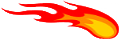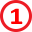﻿ 5. Detailed description of the Actions > 5.15. Operational Research > 5.15.2. KNN (High-Speed action)

# 5.15.2. KNN (High-Speedaction)

Icon:Function: KNN

Property window:Short description:

Solves the K-Nearest-Neighbor problem.

Long Description:

TheKNN Action is typically used for the following two use cases:

1. Data imputation

Let’s assume that you have a customer database that contains many numerical features (such as “age”, “revenue”, “Number of Children”, “Number of Cars”, etc.). So that each customer can be represented by a dot in a space of dimension D (D=number of numerical features inside your customer database). For some customers, some features are missing. You want to “guess” the missing features of a customer Xi by looking at (e.g. most of the time: by “averaging”) the characteristics of the k closests neighbors from the customer Xi. TheKNN Action finds which customers are actually these k neighbors.

You’ll have an Anatella graph such as this one:..where we have:

Parameter P1: The number of neighbor(s) k that you want to find.

Parameter P2: The distance definition. You can choose between:…where we used:Earth-distance in Km (taking into account the curvature of the Earth). X and Y must be GPS coordinates in decimal degree (typically found using thegeocode Action).

Parameter P3: A primary key that uniquely identifies the different customers.

Parameter P4: The variables to include inside the distance computations. The dimention D of the search space is defined by the number of variables given in Parameter P4.

Parameters P5 and P6 are not used in the current use case (see the next use case about “shop assignment” to have more information about these two parameters). To summarize:

oParameter P5: A column that contains a 1/0 (true/false) flag. We’ll only compute the K-Nearest-Neighbor for the rows where the flag is 1 (true).

oParameter P6: A column that contains a 1/0 (true/false) flag. The nearest-neighbors given as output of theKNN Action belongs to the set of rows where the flag is 1 (true).Before starting any computation, Anatella load into RAM memory all the required features. To load data faster, Anatella allocates memory by large chunks. The chunk size is given in Parameter P8.

To decrease computation time: Anatella will use several CPU’s in parellel to compute all the distances. The number of CPU used is given in Parameter P7 (-1=max).

Let’s now assume that our customer database contains N customers.

To solve the KNN problem, we could use a “brute-force-approach” algorithm. This algorithm is:

Loop over all customers inside the database:

Each iteration computes the K-nearest-Neighbor for a difference customer Xi

An iteration is:

oCompute the distance from Xi to all the other customers (there are N-1 such distances) and keep the K customers with the smallest distance.

The “brute-force-approach” is a very slow algorithm because its running time is proportional to N² (since there are about N² distances to compute).

When the dimension D of the search space (D=number of numerical features inside your customer database) is above 5 or 6, it’s very difficult to come with an algorithm that is competitive (in terms of speed) with the simple “brute-force-approach” algorithm (when D is small, “R-trees” are a good family of algorithm). This is why the “brute-force-approach” algorithm is still used most of the time in most KNN tools that regularly deals with high dimentional spaces (D>5).

Anatella does not use a “brute-force-approach” algorithm. The algorithm used to solve the KNN problem in Anatella is optimized for high dimentional spaces (D>5). To reduce computation time, Anatella uses the following algorithm/heuristic:

Loop over all customers inside the database:

Each loop iteration computes the K-Nearest-Neighbors for a different customer Xi (i=1,…N)

The loop is executed in parallel on (Parameter P7) CPU’s.

An iteration is:

oSelect quickly a small “candidate neighbor” of Xi.

oCompute all the distances from Xi to all the customers inside the “candidate neighbor” of Xi.

oKeep the K customers in the “candidate neighbor” that have the smallest distance to Xi (these are the K customers that are returned as the K-Nearest-Neighbors of Xi).

oThe (Parameters P9, P10, P11, P12) are controlling the “candidate neighbor” size and shape.

The selection of the “candidate neighbor” includes a random component (the random number generator is controlled using the Parameter P9).

The “candidate neighbor” size is always smaller than (Parameter P11).

The Parameters P10 and P12 are documented in more details inside the Anatella Advanced Guide.

The Parameter P10 controls the shape of the “candidate neighbor”.

Most of the time, the best (i.e. the fastest) value for the Parameters P10 is “1”.

The Parameter P12 controls the size of the “candidate neighbor”. The best value (and the safest value) for the parameter P12 is the maximum value of “1”. A smaller value (e.g. 0.9) has the following effect:

oit leads to a smaller “candidate neighbor” and thus it reduces the computation time (because there are less distances to compute).

oAnatella might find a wrong answer: i.e. a “too small” value for the parameter P12 reduces so much the size of the “candidate neighbor” that we can’t be assured anymore that the K-Nearest-Neighbors of Xi are actually inside the selected “candidate neighbor”. If such disastrous situation occurs, we’ll return a wrong answer: i.e. we won’t return the exact KNN of Xi but an approximation of it.

o

The parameter P12 (and P11) can be used to balance between a smaller computation time and a more precise/exact answer.

The output of theKNN Action is such a table (with K=1 ; only the first 6 rows of the table are shown):The column “ID” contains the “customer-identifier-column” selected using the parameter P3.

The column “This_Idx” is produced by theKNN Action: This column assigns a unique Key-Number to each different output row. This Key-Number is used inside the column “NN_Idx_1” to indicate who are the closest neighbors.

Let’s give some examples:

Let’s look at the first row (“Frank”) of the above table:

On this row, we see “NN_Idx_1”=3: This means that the closest neighbor to “Frank” is the neighbor with “This_Idx”=3: i.e. the closest neighbor to “Frank” is “Cassian” (and the distance between “Frank” and “Cassian” is “NN_Dist_1”=0.119231).

Let’s look at the third row (“David”) of the above table:

On this row, we see “NN_Idx_1”=1: This means that the closest neighbor to “David” is the neighbor with “This_Idx”=1: i.e. the closest neighbor to “David” is “Sabrina” (and the distance between “David” and “Sabrina” is “NN_Dist_1”=1.40924).

2. Shop assignment/Recommendation

Let’s now assume that you have the GPS coordinates of your shops and of your customer’s homes (you can easily compute these coodinates using thegeocode Action).

The objective of this use case is: For each of your customers, you want to compute their 2 closest shops.

We’ll have an Anatella graph such as this one:Parameter P1: K=2: We only want to get the 2 closest shops.

Parameter P2: Earth Distance

Parameter P4: The 2 columns that contains the latitude and the longitude in that order.

Parameter P5: A column that contains a 1/0 (true/false) flag. Anatella only computes the K-Nearest-Neighbor for the rows where this flag is 1 (true). The Parameter P5 is 1 (true) for all the rows that represents our customers.

Parameter P6: A column that contains a 1/0 (true/false) flag. The nearest-neighbors given as output of theKNN Action can only belong to the set of rows where this flag is 1 (true). The Parameter P6 is 1 (true) for all the rows that represents our shops.

The parameters P5 and P6 bassicaly says: “For all our customers, compute the distances to the shops”.

The (advanced) parameters P7 to P12 are identical to the previous use-case.

An example of output

Let’s assume that we have a customer database with 1000 customers.

We’ll have as output from theKNN Action a table such as:This cellmeans that the closest shop to the customer “Customer_997” is the shop “Shops_3” (because the value of the “This_Idx” for the “Shops_3” is 1002). The “Shops_3” is at 0.815545 Km from “Customer_997”.